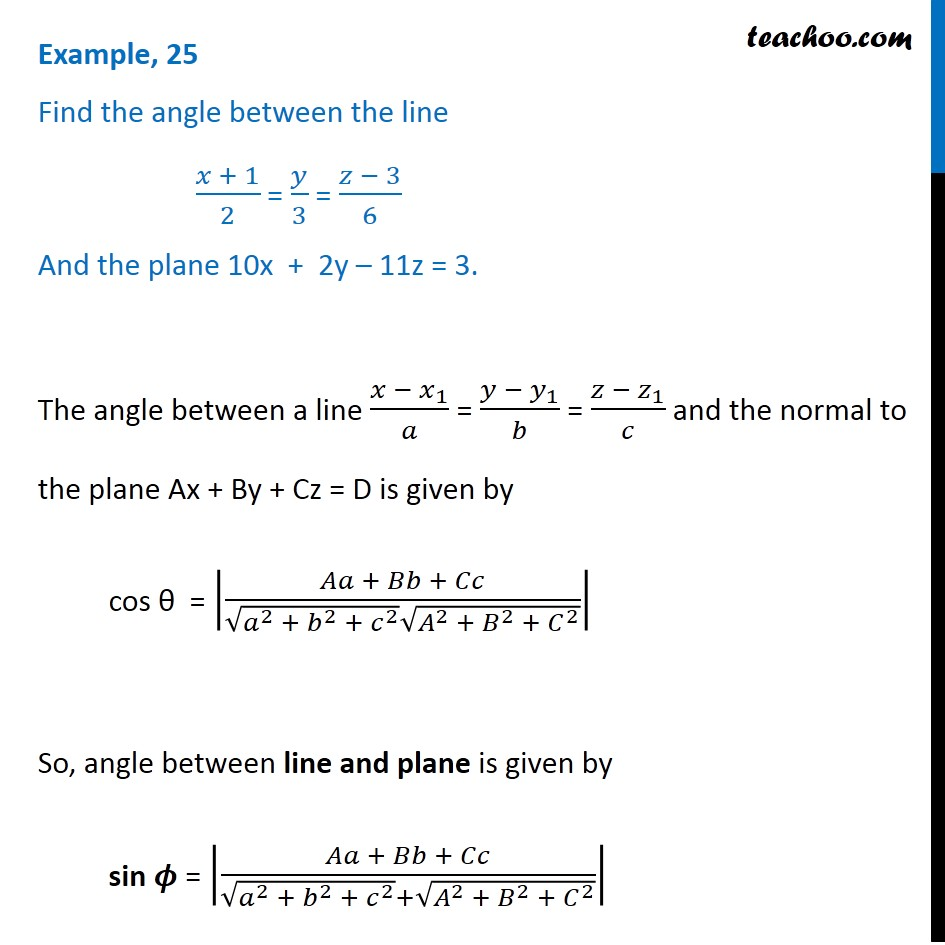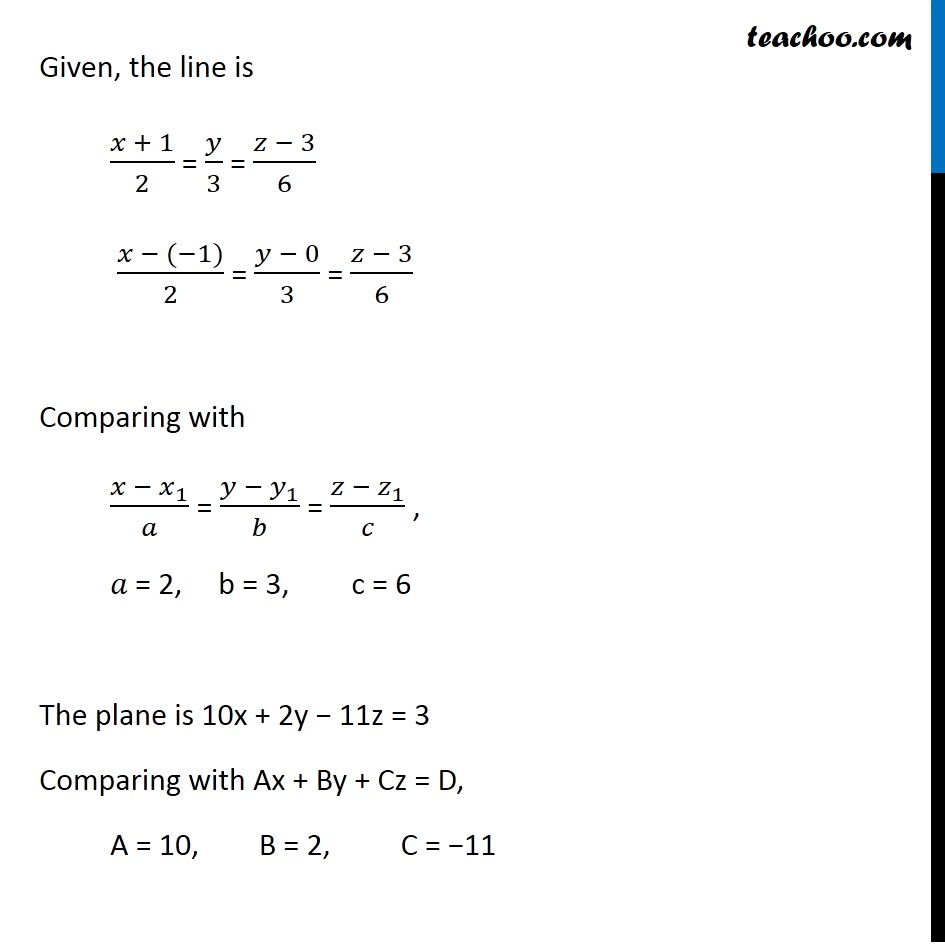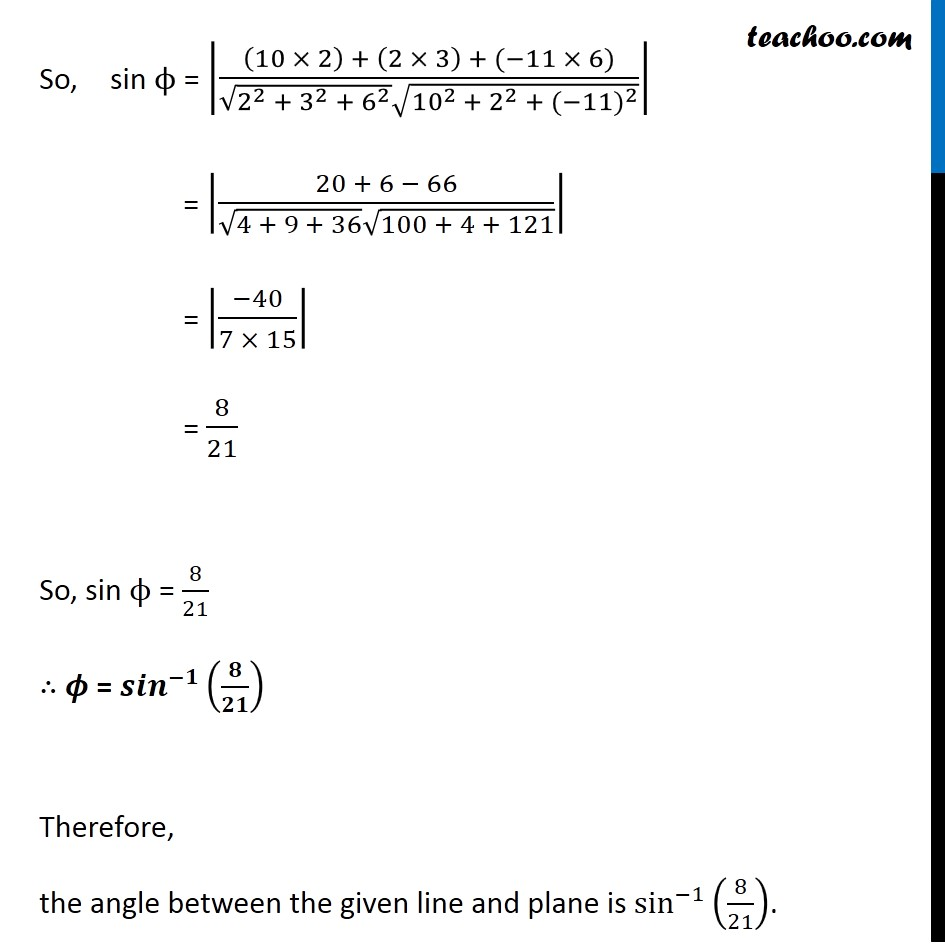Examples

Chapter 11 Class 12 Three Dimensional Geometry
Serial order wiseLearn in your speed, with individual attention - Teachoo Maths 1-on-1 Class

### Transcript

Question 15 Find the angle between the line (𝑥 + 1)/2 = 𝑦/3 = (𝑧 − 3)/6 And the plane 10x + 2y – 11z = 3. The angle between a line (𝑥 − 𝑥_1)/𝑎 = (𝑦 − 𝑦_1)/𝑏 = (𝑧 −〖 𝑧〗_1)/𝑐 and the normal to the plane Ax + By + Cz = D is given by cos θ = |(𝐴𝑎 + 𝐵𝑏 + 𝐶𝑐)/(√(𝑎^2 + 𝑏^2 +〖 𝑐〗^2 ) √(𝐴^2 +〖 𝐵〗^2 +〖 𝐶〗^2 ))| So, angle between line and plane is given by sin 𝝓 = |(𝐴𝑎 + 𝐵𝑏 + 𝐶𝑐)/(√(𝑎^2 + 𝑏^2 + 𝑐^2 )+√(𝐴^2 + 𝐵^2 +〖 𝐶〗^2 ))| Given, the line is (𝑥 + 1)/2 = 𝑦/3 = (𝑧 − 3)/6 (𝑥 − (−1))/2 = (𝑦 − 0)/3 = (𝑧 − 3)/6 Comparing with (𝑥 −〖 𝑥〗_1)/𝑎 = (𝑦 − 𝑦_1)/𝑏 = (𝑧 − 𝑧_1)/𝑐 , 𝑎 = 2, b = 3, c = 6 The plane is 10x + 2y − 11z = 3 Comparing with Ax + By + Cz = D, A = 10, B = 2, C = −11 So, sin ϕ = |((10 × 2) + (2 × 3) + (−11 × 6))/(√(2^2 + 3^2 + 6^2 ) √(〖10〗^(2 )+〖 2〗^2 + 〖(−11)〗^2 ))| = |(20 + 6 − 66)/(√(4 + 9 + 36) √(100 + 4 + 121))| = |(−40)/(7 × 15)| = 8/21 So, sin ϕ = 8/21 ∴ 𝝓 = 〖𝒔𝒊𝒏〗^(−𝟏)⁡(𝟖/𝟐𝟏) Therefore, the angle between the given line and plane is sin^(−1)⁡(8/21).